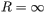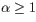# Problem: Energy of a SpacecraftVery far from earth (at ), a spacecraft has run out of fuel and its kinetic energy is zero. If only the gravitational force of the earth were to act on it (i.e., neglect the forces from the sun and other solar system objects), the spacecraft would eventually crash into the earth. The mass of the earth is Me and its radius is Re. Neglect air resistance throughout this problem, since the spacecraft is primarily moving through the near vacuum of space.Part AFind the speed se of the spacecraft when it crashes into the earth.Express the speed in terms of Me, Re , and the universal gravitational constant G.Part BNow find the spacecraft's speed when its distance from the center of the earth is R = αRe, where .Express the speed in terms of se and α.

###### FREE Expert Solution

Part A

Work:

$\overline{)\begin{array}{rcl}{\mathbf{W}}& {\mathbf{=}}& \mathbf{∆}\mathbf{K}\mathbf{E}\end{array}}$

100% (488 ratings)###### Problem Details

Energy of a Spacecraft

Very far from earth (at), a spacecraft has run out of fuel and its kinetic energy is zero. If only the gravitational force of the earth were to act on it (i.e., neglect the forces from the sun and other solar system objects), the spacecraft would eventually crash into the earth. The mass of the earth is Me and its radius is Re. Neglect air resistance throughout this problem, since the spacecraft is primarily moving through the near vacuum of space.

Part A

Find the speed se of the spacecraft when it crashes into the earth.

Express the speed in terms of Me, Re , and the universal gravitational constant G.

Part B

Now find the spacecraft's speed when its distance from the center of the earth is R = αRe, where.

Express the speed in terms of se and α.